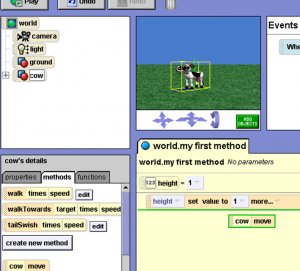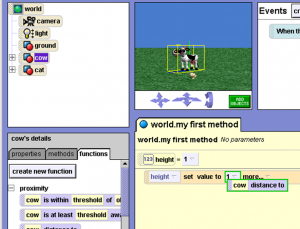## How to Use a Variable and Function in Alice 2

One of the ways that Alice teaches student about returning a value can be seen in how methods, variables and functions work.

## Methods cannot be dragged into variable valuesThis is because , in Alice, a method is an action and does not return a value.

## Functions can be dragged into variable valuesSince functions return a value, they can be dragged into the value of a variable.

So, if you’re ever asked ” e value of this variable to the returned value of a function” then just use this as an example: